﻿ 用MATLAB绘制粒度分布R-R-B线图 Drawing Grain Size Distribution R-R-B Line Diagram by MATLAB

Mine Engineering
Vol.03 No.03(2015), Article ID:15781,5 pages
10.12677/ME.2015.33023

Drawing Grain Size Distribution R-R-B Line Diagram by MATLAB

Fei Li1,2,3,4*

1Tangshan Research Institute, China Coal Technology and Engineering Group, Tangshan Hebei

2Hebei Research Center of Coal Preparation Engineering Technology, Tangshan Hebei

3Tangshan Branch, Tiandi Science & Technology Co. Ltd., Tangshan, Hebei

4Tiandi (Tangshan) Mining Technology Company Limited, Tangshan, Hebei

Email: *aa198524@163.com

Received: Jul. 10th, 2015; accepted: Jul. 27th, 2015; published: Jul. 30th, 2015ABSTRACT

The grain size characteristic equation is the main method to study the grain size characteristics of granular materials, and the R-R equation is used. This paper introduces the current R-R-B drawing, the programming with MATLAB software rendering the new method R-R-B graph is described in detail, and the fitting effect of two methods is experimentally compared which shows the advantages of MATLAB fitting.

Keywords:Size Characteristic, R-R Equation, R-R-B line Diagram, MATLAB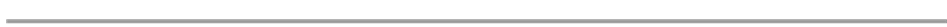1中煤科工集团唐山研究院有限公司，河北 唐山

2河北省煤炭洗选工程技术研究中心，河北 唐山

3天地科技股份有限公司唐山分公司，河北 唐山

4天地(唐山)矿业科技有限公司，河北 唐山

Email: *aa198524@163.com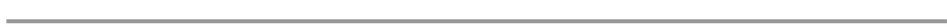1. 引言

2. R-R-B线图的通行画法

R-R方程为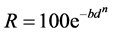(1)

d筛孔尺寸，mm；

b与物料粒度大小有关的参数；

n与物料性质有关的参数。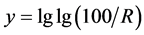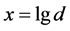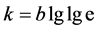，则上式可变换为：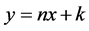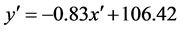(2)

3. 用MATLAB软件画R-R-B图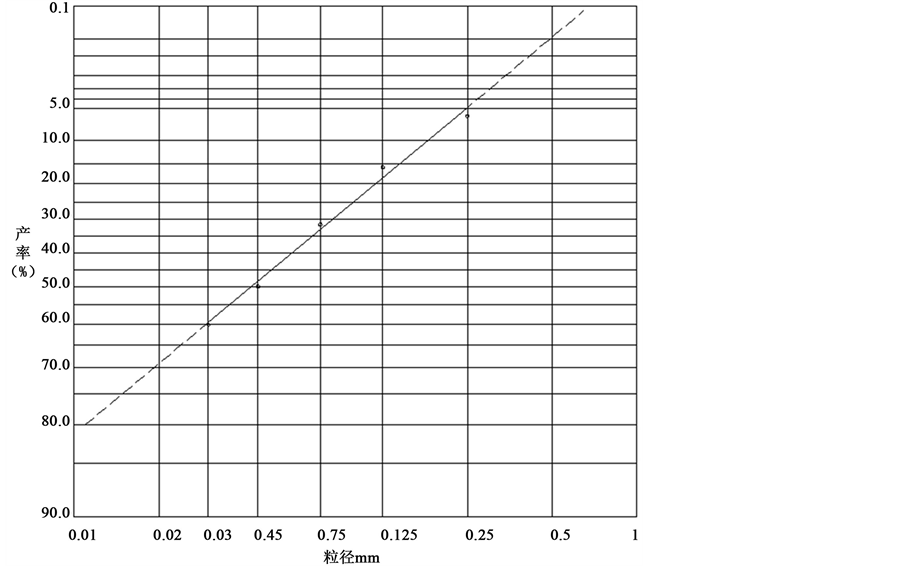Figure 1. R-R-B particle size distribution of linear form

MATLAB是矩阵实验室(Matrix Laboratory)的简称，是美国MathWorks公司出品的商业数学软件，是三大数学软件之一。我们可以用MATLAB软件直接把表1中的X、Y拟合成方程(1)的形式，写出拟合后的方程，并画出拟合曲线，过程简单，其具体编程如下 。

xData =[0.25,0.125,0.075,0.045,0.030]';

yData =[6.05,15.82,31.60,50.01,60.19]';

% Set up fittype and options.

ft = fittype('100*exp(-b*x^n)', 'independent', 'x', 'dependent', 'y');

opts = fitoptions(ft);

opts.Display = 'Off';

opts.Lower = [-Inf -Inf];

opts.StartPoint = [0.913375856139019 0.63235924622541];

opts.Upper = [Inf Inf];

% Fit model to data.

[fitresult, gof] = fit(xData, yData, ft, opts)

% Plot fit with data. figure('Name', 'fit曲线');

x1 = 0:0.01:1;

h = plot(x1,fitresult(x1), xData, yData, '*');

legend(h, 'y vs. x', '拟合曲线', 'Location', 'NorthEast');

% Label axes

xlabel('粒度mm');

ylabel('累积产率%');

grid minor

fitresult =

General model:

fitresult(x) = 100*exp(-b*x^n)

Coefficients (with 95% confidence bounds):

b = 11.08 (6.562, 15.61)

n = 0.8821 (0.7377, 1.026)

gof =

sse: 29.5191

rsquare: 0.9856

dfe: 3

rmse: 3.1368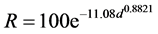4. 两种拟合方法的对比Figure 2. R-R-B line diagram of curve form

5. MATLAB画法的优点

6. 结论

Drawing Grain Size Distribution R-R-B Line Diagram by MATLAB. 矿山工程,03,166-171. doi: 10.12677/ME.2015.33023

1. 1. 王务平, 王微微 (2007) 煤泥(粉)粒度组成对罗辛–拉姆勒粒度特性方程式的验证. 煤炭加工与综合利用, 1, 14- 18.

2. 2. 张荣增, 等 (1996) 水煤浆制浆技术.科学出版社, 北京.

3. 3. 顾民兆 (1982) 粒度分布(R-R-B)线图的由来和应用. 煤矿设计, 2, 19-25.

4. 4. 刘鹏, 焦红光 (2008) 选煤厂煤泥粒度特性的研究. 河南理工大学学报, 5, 582-585.

5. 5. 张建武, 王文霞 (2011) 机械振动的MATLAB处理. 河西学院学报, 2, 106-107.